JMSLTM Numerical Library 7.2.0
com.imsl.math

## Class MinUnconMultiVar

• All Implemented Interfaces:
Serializable, Cloneable

```public class MinUnconMultiVar
extends Object
implements Serializable, Cloneable```
Unconstrained multivariate minimization.

Class `MinUnconMultivar` uses a quasi-Newton method to find the minimum of a function f(x) of n variables. The problem is stated as follows: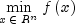Given a starting point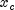, the search direction is computed according to the formula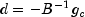where B is a positive definite approximation of the Hessian, and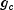is the gradient evaluated at. A line search is then used to find a new point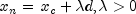such that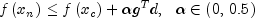Finally, the optimality condition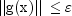whereis a gradient tolerance, is sought.

When optimality is not achieved, B is updated according to the BFGS formula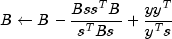where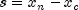and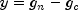. Another search direction is then computed to begin the next iteration. For more details, see Dennis and Schnabel (1983, Appendix A).

In this implementation, the first stopping criterion for `MinUnconMultivar` occurs when the norm of the gradient is less than the given gradient tolerance `gradientTolerance`. The second stopping criterion for `MinUnconMultivar` occurs when the scaled distance between the last two steps is less than the step tolerance `stepTolerance`.

Since by default, a finite-difference method is used to estimate the gradient for some single precision calculations, an inaccurate estimate of the gradient may cause the algorithm to terminate at a noncritical point. Supply the gradient for a more accurate gradient evaluation (`setGradient`).

The user may also choose to supply the Hessian. If, during intermediate calculations, the user supplied Hessian becomes non-symmetric positive definite, then the algorithm will resort to using the default approximate Hessian.

Finite-difference Example, Gradient Example, Hessian Example, Serialized Form
• ### Nested Class Summary

Nested Classes
Modifier and Type Class and Description
`static class ` `MinUnconMultiVar.ApproximateMinimumException`
Scaled step tolerance satisfied; the current point may be an approximate local solution, or the algorithm is making very slow progress and is not near a solution, or the scaled step tolerance is too big.
`static class ` `MinUnconMultiVar.FalseConvergenceException`
False convergence error; the iterates appear to be converging to a noncritical point.
`static interface ` `MinUnconMultiVar.Function`
Public interface for the user supplied function to the `MinUnconMultiVar` object.
`static interface ` `MinUnconMultiVar.Gradient`
Public interface for the user supplied gradient to the `MinUnconMultiVar` object.
`static interface ` `MinUnconMultiVar.Hessian`
Public interface for the user supplied Hessian to the `MinUnconMultiVar` object.
`static class ` `MinUnconMultiVar.MaxIterationsException`
Maximum number of iterations exceeded.
`static class ` `MinUnconMultiVar.UnboundedBelowException`
Five consecutive steps of the maximum allowable stepsize have been taken, either the function is unbounded below, or has a finite asymptote in some direction or the maximum allowable step size is too small.
• ### Constructor Summary

Constructors
Constructor and Description
`MinUnconMultiVar(int n)`
Unconstrained minimum constructor for a function of n variables of type `double`.
• ### Method Summary

Methods
Modifier and Type Method and Description
`double[]` `computeMin(MinUnconMultiVar.Function F)`
Return the minimum point of a function of n variables of type `double` using a finite-difference gradient or using a user-supplied gradient.
`int` `getErrorStatus()`
Returns the non-fatal error status.
`int` `getIterations()`
Returns the number of iterations used to compute a minimum.
`int` `getNumberOfThreads()`
Returns the number of `java.lang.Thread` instances used for parallel processing.
`void` `setDigits(double fdigit)`
Set the number of good digits in the function.
`void` `setFscale(double fscale)`
Set the function scaling value for scaling the gradient.
`void` `setGradientTolerance(double gradientTolerance)`
`void` `setGuess(double[] xguess)`
Set the initial guess of the minimum point of the input function.
`void` `setIhess(int ihess)`
Set the Hessian initialization parameter.
`void` `setMaximumStepsize(double maximumStepsize)`
Set the maximum allowable stepsize to use.
`void` `setMaxIterations(int maxIterations)`
Set the maximum number of iterations allowed.
`void` `setNumberOfThreads(int numberOfThreads)`
Sets the number of `java.lang.Thread` instances to be used for parallel processing.
`void` `setStepTolerance(double stepTolerance)`
Set the scaled step tolerance to use when changing x.
`void` `setXscale(double[] xscale)`
Set the diagonal scaling matrix for the variables.
• ### Methods inherited from class java.lang.Object

`clone, equals, finalize, getClass, hashCode, notify, notifyAll, toString, wait, wait, wait`
• ### Constructor Detail

• #### MinUnconMultiVar

`public MinUnconMultiVar(int n)`
Unconstrained minimum constructor for a function of n variables of type `double`.
Parameters:
`n` - An `int` scalar value which defines the number of variables of the function whose minimum is to be found.
• ### Method Detail

• #### computeMin

```public double[] computeMin(MinUnconMultiVar.Function F)
throws MinUnconMultiVar.FalseConvergenceException,
MinUnconMultiVar.MaxIterationsException,
MinUnconMultiVar.UnboundedBelowException```
Return the minimum point of a function of n variables of type `double` using a finite-difference gradient or using a user-supplied gradient.
Parameters:
`F` - defines the function whose minimum is to be found. `F` can be used to supply a gradient of the function. If `F` implements `Gradient` then the user-supplied gradient is used. Otherwise, an attempt to find the minimum is made using a finite-difference gradient. If F implements `Hessian` then the user-supplied gradient and Hessian are used. Otherwise, an approximate Hessian is used during computation.
Returns:
a `double` array containing the point at which the minimum of the input function occurs.
Throws:
`MinUnconMultiVar.FalseConvergenceException`
`MinUnconMultiVar.MaxIterationsException`
`MinUnconMultiVar.UnboundedBelowException`
• #### getErrorStatus

`public int getErrorStatus()`
Returns the non-fatal error status.
Returns:
an `int` specifying the non-fatal error status:  Status Meaning 1 The last global step failed to locate a lower point than the current x value. The current x may be an approximate local minimizer and no more accuracy is possible or the step tolerance may be too large. 2 Relative function convergence; both the actual and predicted relative reductions in the function are less than or equal to the relative function convergence tolerance. 3 Scaled step tolerance satisfied; the current point may be an approximate local solution, or the algorithm is making very slow progress and is not near a solution, or the step tolerance is too big.

• #### getIterations

`public int getIterations()`
Returns the number of iterations used to compute a minimum.
Returns:
an `int` specifying the number of iterations used to compute the minimum.

`public int getNumberOfThreads()`
Returns the number of `java.lang.Thread` instances used for parallel processing.
Returns:
an `int` containing the number of `java.lang.Thread` instances used for parallel processing.
• #### setDigits

`public void setDigits(double fdigit)`
Set the number of good digits in the function. If this member function is not called, fdigit is set to 15.0.
Parameters:
`fdigit` - a `double` scalar value specifying the number of good digits in the user supplied function
Throws:
`IllegalArgumentException` - is thrown if `fdigit` is less than or equal to 0
• #### setFscale

`public void setFscale(double fscale)`
Set the function scaling value for scaling the gradient. If this member function is not called, the value of this scalar is set to 1.0.
Parameters:
`fscale` - a `double` scalar specifying the function scaling value for scaling the gradient
Throws:
`IllegalArgumentException` - is thrown if `fscale` is less than or equal to 0.

`public void setGradientTolerance(double gradientTolerance)`
Sets the gradient tolerance. This first stopping criterion for this optimizer is that the norm of the gradient be less than the gradient tolerance. If this member function is not called, the cube root of machine precision squared is used to compute the gradient.
Parameters:
`gradientTolerance` - a `double` specifying the gradient tolerance used to compute the gradient
Throws:
`IllegalArgumentException` - is thrown if gradientTolerance is less than or equal to 0
• #### setGuess

`public void setGuess(double[] xguess)`
Set the initial guess of the minimum point of the input function. If this member function is not called, the elements of this array are set to 0.0..
Parameters:
`xguess` - a `double` array specifying the initial guess of the minimum point of the input function
• #### setIhess

`public void setIhess(int ihess)`
Set the Hessian initialization parameter. If this member function is not called, ihess is set to 0.0 and the Hessian is initialized to the identity matrix. If this member function is called and ihess is set to anything other than 0.0, the Hessian is initialized to the diagonal matrix containing max(abs(f(xguess)),fscale)*xscale*xscale
Parameters:
`ihess` - an `int` scalar value specifying the Hessian initialization parameter. If `ihess` = 0.0 the Hessian is initialized to the identity matrix. Otherwise, the Hessian is initialized to the diagonal matrix containing max(abs(f(xguess)),fscale)*xscale*xscale where xguess is the initial guess of the computed solution and xscale is the scaling vector for the variables.
• #### setMaximumStepsize

`public void setMaximumStepsize(double maximumStepsize)`
Set the maximum allowable stepsize to use. If this member function is not called, maximum stepsize is set to a default value based on a scaled xguess.
Parameters:
`maximumStepsize` - a nonnegative `double` value specifying the maximum allowable stepsize
Throws:
`IllegalArgumentException` - is thrown if maximumStepsize is less than or equal to 0
• #### setMaxIterations

`public void setMaxIterations(int maxIterations)`
Set the maximum number of iterations allowed. If this member function is not called, the maximum number of iterations is set to 100.
Parameters:
`maxIterations` - an `int` specifying the maximum number of iterations allowed
Throws:
`IllegalArgumentException` - is thrown if maxIterations is less than or equal to 0

`public void setNumberOfThreads(int numberOfThreads)`
Sets the number of `java.lang.Thread` instances to be used for parallel processing.
Parameters:
`numberOfThreads` - an `int` specifying the number of `java.lang.Thread` instances to be used for parallel processing. If `numberOfThreads` is greater than 1, then interface `Function.f` is evaluated in parallel and `Function.f` must be thread-safe. Otherwise, unexpected behavior can occur.

Default: `numberOfThreads` = 1.

• #### setStepTolerance

`public void setStepTolerance(double stepTolerance)`
Set the scaled step tolerance to use when changing x. If this member function is not called, the scaled step tolerance is set to 3.66685e-11.

The second stopping criterion for this optimizer is that the scaled distance between the last two steps be less than the step tolerance.

Parameters:
`stepTolerance` - a `double` scalar value specifying the scaled step tolerance. The i-th component of the scaled step between two points x and y is computed as abs(x(i)-y(i))/max(abs(x(i)),1/xscale(i)) where xscale is the scaling vector for the variables.
Throws:
`IllegalArgumentException` - is thrown if stepTolerance is less than or equal to 0
• #### setXscale

`public void setXscale(double[] xscale)`
Set the diagonal scaling matrix for the variables. If this member function is not called, the elements of this array are set to 1.0..
Parameters:
`xscale` - a `double` array specifying the diagonal scaling matrix for the variables
Throws:
`IllegalArgumentException` - is thrown if any of the elements of xscale is less than or equal to 0
JMSLTM Numerical Library 7.2.0